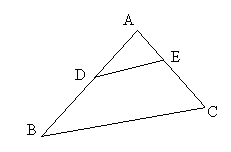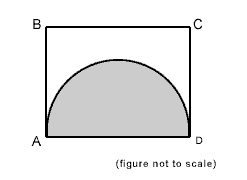50万+已关注

# SAT数学练习题整理9

2019-02-07 17:26:00来源：网络

新东方在线SAT频道为大家带来SAT数学练习题整理9一文，希望对大家SAT备考有所帮助。更多精彩尽请关注新东方在线SAT频道!

1. (3 x 104) + (2 x 102) + (4 x 10) =

A. 302400

B. 32400

C. 30240

D. 3240

E. 324

2. Andy solves problems 74 to 125 inclusive in a Math exercise. How many problems does he solve?

A. 53

B. 52

C. 51

D. 50

E. 49

3. If x and y are integers, and 3x + 2y = 13, which of the following could be the value of y ?

A. 0

B. 1

C. 2

D. 3

E. 44. In triangle ABC, AD = DB , DE is parallel to BC, and the area of triangle ABC is 40. What is the area of triangle ADE ?

A. 10

B. 15

C. 20

D. 30

E. it cannot be determined from the information given

5. If n > 0 , which of the following must be true?

I n2 > 1

II n - n2 < 0

III 2n - 1 > 0

A. I only

B. II only

C. III only

D. I and II only

E. none

6. If the slope of a line is and the y-intercept is 3, what is the x-intercept of the same line?

A. 6

B. 3/2

C. 0

D. -2/3

E. -6

7. 6 people meet for a business lunch. Each person shakes hands once with each other person present. How many handshakes take place?

A. 30

B. 21

C. 18

D. 15

E. 10

8. If x2 - y2 = 55, and x - y = 11, then y =

A. 8

B. 5

C. 3

D. -8

E. -3

9. In a sports club with 30 members, 17 play badminton and 19 play tennis and 2 do not play either. How many members play both badminton and tennis?

A. 7

B. 8

C. 9

D. 10

E. 1110. Rectangle ABCD has a perimeter of 26. The half circle with diameter AD has an area of 8π. What is the perimeter of the part of the figure that is not shaded?

A. 26 + 4π

B. 18 + 8π

C. 18 + 4π

D. 14 + 4π

E. 14 + 2π

Explanation:

2 x 104 = 30,000; 2 x 102 = 200; 4 x 10 = 40

The total is 30,240

Explanation:

To find how many problems in the series we need to take the difference and add one.

125 - 74 = 51; 51 + 1 =52

Explanation:

Substitute the given values for y and check whether you get an integer value for x.

For example, using 0 we get 3x = 13; x = 13/3 which is not a whole number.

The right answer is 2, since 3x + 2(2) = 13; 3x = 13 - 4 = 9; x = 9/3 = 3.

Explanation:

The big triangle ABC is similar to the small triangle ADE because their bases are parallel. If corresponding side of two similar triangles are known the ratio of the areas is also known. In this case, let AD be one unit, then AB is 2 units (given that AB = AD + DB). The ratios of the sides is 1 : 2. The ratio of the areas will be (1)2 : (2)2 ; 1 : 4

Since the big triangle has area 40, using the ratio, the small has area 10.

Explanation:

Given that n is positive, it could be a positive fraction, 1, or a fraction of whole number greater than 1.

If n = 1, then case I is not true since n2 = 1

Likewise in II if n = 1, n - n2 = 0, and the statement is not true.

In III, if n = , then 2n - 1 = 0, and again the statement is incorrect.

Explanation:

The equation for a straight line is y = mx + c, where m = slope and c = y-intercept.

Putting the given values in this equation we have y = x/2 + 3

The x-intercept occurs where y = 0. Thus, 0 = x/2 + 3 ; -3 = x/2 ; -6 =x

Explanation:

Imagine the first person of the six. He or she will have to shake hands with each of the other 5. Now turn to the second person. He or she will have to shake with the other five, but he she has already shaken with the first person. This means 4 new handshakes. The third person will have to shake with 5 - 2 = 3 people, and so on. Total handshakes = 5 + 4 + 3 + 2 + 1 = 15

Explanation:

x2 - y2 can be expressed as (x + y)(x - y); since x - y =11 we can write

(x + y)11 = 55; therefore x + y = 5

Adding the two equations x + y = 5 and x - y = 11 we get

2x = 16; x = 8

Therefore 8 - y = 11; y = -3

Explanation:

Since 2 do not play either, there are 28 members who play one sport or the other. Let the number who play both be n.

Total (28) will be made up of only badminton players (17 - n), plus only tennis (19 - n) and those who play both (n).

28 = (17 - n) + (19 - n) + n

28 = 36 - n ; n = 8

Explanation:

The total perimeter of the un-shaded part is made up of three sides of the rectangle and the perimeter of the half-circle.

The area of a half circle = π r2

8 π = π r2; therefore r = 4

The perimeter of the half circle is 8 π = 4 π

The diameter of the circle (8) = the length of the rectangle.

Total perimeter of the rectangle = 26

Three sides measure 26 - 8 = 18 Ans. 18 + 4π

以上就为大家整理的“SAT数学练习题整理9”，更多精彩内容，请关注新东方在线SAT频道。

SATII精讲班
SAT名师一对一

SAT考生必修课
SAT考生，高效提分，安心之选

• 课时 : 91琚晋蓉张霄

￥2680

18人 已报到

免费试听

• 1
• 2
• 3
• 4
• 5
• 6
• 7
• 8
• 9
• 10